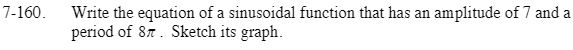Home > CCA2 > Chapter 7 > Lesson 7.2.4 > Problem7-160

7-160.

Write the equation of a sinusoidal function that has an amplitude of 7 and a period of 8π. Sketch its graph. Homework Help ✎In the general equation y = a · sin[b(xh)] + k, the parameter a is the amplitude and b is the number of cycles in 2π.

See the Math Notes box for this lesson (7.2.4) and problem 7-159.

Test your equation on a graphing calculator to see if it meets the given criteria.